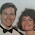## Sunday, March 13, 2016

### Discovering Pi with Google Sheets

Many concepts in mathematics can be discovered by students rather than simply being told. Sometimes this is called inquiry learning.

Math is full of patterns and predicable relationships. When students discover these patterns themselves and the draw conclusions they will understand and remember the concepts so much better than if we were to just tell them the information.

One example of this is the concept of pi. It is very easy for us to say "Pi is 3.14" or "Pi is the ratio of the circumference of a circle to the diameter of the same circle" but that is just giving the students information, not helping them discover and truly understand what pi means.

One way to do this is an activity that uses Google Sheets and allows the students to measure real life circular objects, examine some calculations, look for patterns, and discover what pi is and what it means.

See below for a spreadsheet template you can copy and use for this activity, as well as a short tutorial video and detailed directions on how to do the project.

Tutorial Video (10 minutes)

The spreadsheet to be used for this activity can be found at the link below. Simply click the link to get your own editable copy of the spreadsheet.

Activity Directions

Below are the steps for this activity:
1. Each student will need a circular object, such as a can, lid, frisbee, plate, or such. For best results the items should be a variety of sizes.
2. Students will also need a tape measurer (one that can easily wrap around the circular object).
3. First each student should measure the distance around their circular object.
4. Next each student should measure the distance across their circular object, being careful to go directly through the middle of the circle.
5. The students should then record their measurements on the spreadsheet tab titled "Data"
6. The spreadsheet will automatically calculate the ratio of the circumference to the diameter for each student. (Note: If you want, you can hide that column or add it later so that you can first discuss with the students what patterns they see.)
7. The spreadsheet will also calculate an average of all the ratios from all the students.
8. You can also switch over to the tab titled "Chart" where a scatter plot of the data will be generated. This is another good option for the students to look for a relationship between the circumference and diameter.
9. The students should examine their ratios, the average ration, and the scatter plot to see if they notice any patterns or relationships.
10. Finally switch over to the spreadsheet tab titled "Pi" to compare how close the students' average ratio is to the real value of pi.

By doing this activity students will discover that no matter how big or small a circle is, the distance around the circle is always a little more than three times as much as the distance across. That is, the circumference is always pi times more than the diameter.

This activity also checks off two of the 4 C's of Education:
• Collaboration - the students are working together as a class to collect and analyze data.
• Critical Thinking - instead of just telling our students what pi is, we are giving them the opportunity to study data, look for patterns, and draw their own conclusions.
For more details on the 4 C's of Education see my blog post and video here.

Post by Eric Curts. Bring me to your school, organization, or conference with over 50 PD sessions to choose from. Connect with me on Twitter at twitter.com/ericcurts and on Google+ at plus.google.com/+EricCurts1

1.2.